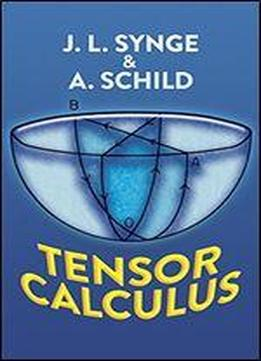# Tensor Calculus by John Lighton Synge / 1978 / English / PDF

"This book is an excellent classroom text, since it is clearly written, contains numerous problems and exercises, and at the end of each chapter has a summary of the significant results of the chapter." Quarterly of Applied Mathematics. Fundamental introduction for beginning student of absolute differential calculus and for those interested in applications of tensor calculus to mathematical physics and engineering. Topics include spaces and tensors basic operations in Riemannian space, curvature of space, special types of space, relative tensors, ideas of volume, and more.

views: 463